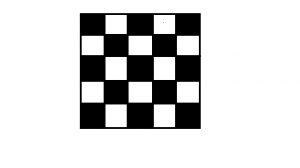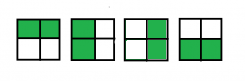# Ways to place K bishops on an N×N chessboard so that no two attack

Given two integers N and K, the task is to find the number of ways to place K bishops on an N × N chessboard so that no two bishops attack each other.

Here is an example for a 5×5 chessboard.Examples:

Input: N = 2, K = 2
Output: 4
The different ways to place 2 bishops in a 2 * 2 chessboard are :Input: N = 4, K = 3
Output: 232

## Recommended: Please try your approach on {IDE} first, before moving on to the solution.

Approach: This problem can be solved using dynamic programming.

• Let dp[i][j] denote the number of ways to place j bishops on diagonals with indices up to i which have the same color as diagonal i. Then i = 1…2N-1 and j = 0…K.
• We can calculate dp[i][j] using only values of dp[i-2] (we subtract 2 because we only consider diagonals of the same color as i). There are two ways to get dp[i][j]. Either we place all j bishops on previous diagonals: then there are dp[i-2][j] ways to achieve this. Or we place one bishop on diagonal i and j-1 bishops on previous diagonals. The number of ways to do this equals the number of squares in diagonal i – (j – 1), because each of j-1 bishops placed on previous diagonals will block one square on the current diagonal.
• The base case is simple: dp[i] = 1, dp = 1.
• Once we have calculated all values of dp[i][j], the answer can be obtained as follows: consider all possible numbers of bishops placed on black diagonals i=0…K, with corresponding numbers of bishops on white diagonals K-i. The bishops placed on black and white diagonals never attack each other, so the placements can be done independently. The index of the last black diagonal is 2N-1, the last white one is 2N-2. For each i we add dp[2N-1][i] * dp[2N-2][K-i] to the answer.

Below is the implementation of the above approach:

## C++

 `// CPP implementation of the approach ` `#include ` `using` `namespace` `std; ` ` `  `// returns the number of squares in diagonal i ` `int` `squares(``int` `i) ` `{ ` `     `  `    ``if` `((i & 1) == 1) ` `        ``return` `i / 4 * 2 + 1; ` `    ``else` `        ``return` `(i - 1) / 4 * 2 + 2; ` `} ` ` `  `// returns the number of ways to fill a ` `// n * n chessboard with k bishops so ` `// that no two bishops attack each other. ` `long` `bishop_placements(``int` `n, ``int` `k) ` `{ ` `    ``// return 0 if the number of valid places to be ` `    ``// filled is less than the number of bishops ` `    ``if` `(k > 2 * n - 1) ` `        ``return` `0; ` ` `  `    ``// dp table to store the values ` `    ``long` `dp[n * 2][k + 1]; ` ` `  `    ``// Setting the base conditions ` `    ``for``(``int` `i = 0; i < n * 2; i++) ` `    ``{ ` `        ``for``(``int` `j = 0; j < k + 1; j++) ` `        ``{ ` `            ``dp[i][j] = 0; ` `        ``} ` `     `  `    ``} ` `    ``for` `(``int` `i = 0; i < n * 2; i++) ` `        ``dp[i] = 1; ` `    ``dp = 1; ` ` `  `    ``// calculate the required number of ways ` `    ``for` `(``int` `i = 2; i < n * 2; i++)  ` `    ``{ ` `        ``for` `(``int` `j = 1; j <= k; j++) ` `        ``{ ` `            ``dp[i][j] = dp[i - 2][j]  ` `                    ``+ dp[i - 2][j - 1] * (squares(i) - j + 1); ` ` `  `        ``} ` `    ``} ` ` `  `    ``// stores the answer ` `    ``long` `ans = 0; ` `    ``for` `(``int` `i = 0; i <= k; i++) ` `    ``{ ` `        ``ans += dp[n * 2 - 1][i] * dp[n * 2 - 2][k - i]; ` `    ``} ` ` `  `    ``return` `ans; ` `} ` ` `  `// Driver code ` `int` `main() ` `{ ` `    ``int` `n = 2; ` `    ``int` `k = 2; ` `    ``long` `ans = bishop_placements(n, k); ` `    ``cout << (ans); ` `} ` ` `  `// This code is contributed by Rajput-Ji `

## Java

 `// Java implementation of the approach ` ` `  `class` `GFG { ` ` `  `    ``// returns the number of squares in diagonal i ` `    ``static` `int` `squares(``int` `i) ` `    ``{ ` `        ``if` `((i & ``1``) == ``1``) ` `            ``return` `i / ``4` `* ``2` `+ ``1``; ` `        ``else` `            ``return` `(i - ``1``) / ``4` `* ``2` `+ ``2``; ` `    ``} ` ` `  `    ``// returns the number of ways to fill a ` `    ``// n * n chessboard with k bishops so ` `    ``// that no two bishops attack each other. ` `    ``static` `long` `bishop_placements(``int` `n, ``int` `k) ` `    ``{ ` `        ``// return 0 if the number of valid places to be ` `        ``// filled is less than the number of bishops ` `        ``if` `(k > ``2` `* n - ``1``) ` `            ``return` `0``; ` ` `  `        ``// dp table to store the values ` `        ``long``[][] dp = ``new` `long``[n * ``2``][k + ``1``]; ` ` `  `        ``// Setting the base conditions ` `        ``for` `(``int` `i = ``0``; i < n * ``2``; i++) ` `            ``dp[i][``0``] = ``1``; ` `        ``dp[``1``][``1``] = ``1``; ` ` `  `        ``// calculate the required number of ways ` `        ``for` `(``int` `i = ``2``; i < n * ``2``; i++) { ` `            ``for` `(``int` `j = ``1``; j <= k; j++) ` `                ``dp[i][j]  ` `                    ``= dp[i - ``2``][j]  ` `                        ``+ dp[i - ``2``][j - ``1``] * (squares(i) - j + ``1``); ` `        ``} ` ` `  `        ``// stores the answer ` `        ``long` `ans = ``0``; ` `        ``for` `(``int` `i = ``0``; i <= k; i++) { ` `            ``ans += dp[n * ``2` `- ``1``][i] * dp[n * ``2` `- ``2``][k - i]; ` `        ``} ` ` `  `        ``return` `ans; ` `    ``} ` ` `  `    ``// Driver code ` `    ``public` `static` `void` `main(String[] args) ` `    ``{ ` `        ``int` `n = ``2``; ` `        ``int` `k = ``2``; ` `        ``long` `ans = bishop_placements(n, k); ` `        ``System.out.println(ans); ` `    ``} ` `} `

## Python3

 `# Python 3 implementation of the approach ` ` `  `# returns the number of squares in  ` `# diagonal i ` `def` `squares(i): ` `    ``if` `((i & ``1``) ``=``=` `1``): ` `        ``return` `int``(i ``/` `4``) ``*` `2` `+` `1` `    ``else``: ` `        ``return` `int``((i ``-` `1``) ``/` `4``) ``*` `2` `+` `2` ` `  `# returns the number of ways to fill a ` `# n * n chessboard with k bishops so ` `# that no two bishops attack each other. ` `def` `bishop_placements(n, k): ` `     `  `    ``# return 0 if the number of valid places  ` `    ``# to be filled is less than the number  ` `    ``# of bishops ` `    ``if` `(k > ``2` `*` `n ``-` `1``): ` `        ``return` `0` ` `  `    ``# dp table to store the values ` `    ``dp ``=` `[[``0` `for` `i ``in` `range``(k ``+` `1``)]  ` `             ``for` `i ``in` `range``(n ``*` `2``)] ` ` `  `    ``# Setting the base conditions ` `    ``for` `i ``in` `range``(n ``*` `2``): ` `        ``dp[i][``0``] ``=` `1` `         `  `    ``dp[``1``][``1``] ``=` `1` ` `  `    ``# calculate the required number of ways ` `    ``for` `i ``in` `range``(``2``, n ``*` `2``, ``1``): ` `        ``for` `j ``in` `range``(``1``, k ``+` `1``, ``1``): ` `            ``dp[i][j] ``=` `(dp[i ``-` `2``][j] ``+`  `                        ``dp[i ``-` `2``][j ``-` `1``] ``*` `                       ``(squares(i) ``-` `j ``+` `1``)) ` ` `  `    ``# stores the answer ` `    ``ans ``=` `0` `    ``for` `i ``in` `range``(``0``, k ``+` `1``, ``1``): ` `        ``ans ``+``=` `(dp[n ``*` `2` `-` `1``][i] ``*`  `                ``dp[n ``*` `2` `-` `2``][k ``-` `i]) ` ` `  `    ``return` `ans ` ` `  `# Driver code ` `if` `__name__ ``=``=` `'__main__'``: ` `    ``n ``=` `2` `    ``k ``=` `2` `    ``ans ``=` `bishop_placements(n, k) ` `    ``print``(ans) ` ` `  `# This code is contributed by ` `# Sanjit_Prasad `

## C#

 `// C# implementation of the approach  ` `using` `System; ` ` `  `class` `GFG ` `{ ` ` `  `// returns the number of squares  ` `// in diagonal i  ` `static` `int` `squares(``int` `i)  ` `{  ` `    ``if` `((i & 1) == 1)  ` `        ``return` `i / 4 * 2 + 1;  ` `    ``else` `        ``return` `(i - 1) / 4 * 2 + 2;  ` `}  ` ` `  `// returns the number of ways to fill a  ` `// n * n chessboard with k bishops so  ` `// that no two bishops attack each other.  ` `static` `long` `bishop_placements(``int` `n, ``int` `k)  ` `{  ` `    ``// return 0 if the number of valid  ` `    ``// places to be filled is less than ` `    ``// the number of bishops  ` `    ``if` `(k > 2 * n - 1)  ` `        ``return` `0;  ` ` `  `    ``// dp table to store the values  ` `    ``long``[,] dp = ``new` `long``[n * 2, k + 1];  ` ` `  `    ``// Setting the base conditions  ` `    ``for` `(``int` `i = 0; i < n * 2; i++)  ` `        ``dp[i, 0] = 1;  ` `    ``dp[1, 1] = 1;  ` ` `  `    ``// calculate the required ` `    ``// number of ways  ` `    ``for` `(``int` `i = 2; i < n * 2; i++)  ` `    ``{  ` `        ``for` `(``int` `j = 1; j <= k; j++)  ` `            ``dp[i, j] = dp[i - 2, j] +  ` `                       ``dp[i - 2, j - 1] *  ` `                        ``(squares(i) - j + 1);  ` `    ``}  ` ` `  `    ``// stores the answer  ` `    ``long` `ans = 0;  ` `    ``for` `(``int` `i = 0; i <= k; i++)  ` `    ``{  ` `        ``ans += dp[n * 2 - 1, i] *  ` `               ``dp[n * 2 - 2, k - i];  ` `    ``}  ` ` `  `    ``return` `ans;  ` `}  ` ` `  `// Driver code  ` `static` `public` `void` `Main () ` `{ ` `    ``int` `n = 2;  ` `    ``int` `k = 2;  ` `    ``long` `ans = bishop_placements(n, k);  ` `    ``Console.WriteLine(ans);  ` `} ` `} ` ` `  `// This code is contributed by akt_mit `

## PHP

 ` 2 * ``\$n` `- 1) ` `        ``return` `0; ` ` `  `    ``// dp table to store the values ` `    ``\$dp` `= ``array_fill``(0, ``\$n` `* 2, ` `          ``array_fill``(0, ``\$k` `+ 1, NULL)); ` ` `  `    ``// Setting the base conditions ` `    ``for` `(``\$i` `= 0; ``\$i` `< ``\$n` `* 2; ``\$i``++) ` `        ``\$dp``[``\$i``] = 1; ` `    ``\$dp`` = 1; ` ` `  `    ``// calculate the required number of ways ` `    ``for` `(``\$i` `= 2; ``\$i` `< ``\$n` `* 2; ``\$i``++) ` `    ``{ ` `        ``for` `(``\$j` `= 1; ``\$j` `<= ``\$k``; ``\$j``++) ` `            ``\$dp``[``\$i``][``\$j``] = ``\$dp``[``\$i` `- 2][``\$j``] +  ` `                          ``\$dp``[``\$i` `- 2][``\$j` `- 1] *  ` `                             ``(squares(``\$i``) - ``\$j` `+ 1); ` `    ``} ` ` `  `    ``// stores the answer ` `    ``\$ans` `= 0; ` `    ``for` `(``\$i` `= 0; ``\$i` `<= ``\$k``; ``\$i``++) ` `    ``{ ` `        ``\$ans` `+= ``\$dp``[``\$n` `* 2 - 1][``\$i``] * ` `                ``\$dp``[``\$n` `* 2 - 2][``\$k` `- ``\$i``]; ` `    ``} ` ` `  `    ``return` `\$ans``; ` `} ` ` `  `// Driver code ` `\$n` `= 2; ` `\$k` `= 2; ` `\$ans` `= bishop_placements(``\$n``, ``\$k``); ` `echo` `\$ans``; ` ` `  `// This code is contributed by ita_c ` `?> `

Output:

```4
```

GeeksforGeeks has prepared a complete interview preparation course with premium videos, theory, practice problems, TA support and many more features. Please refer Placement 100 for details

My Personal Notes arrow_drop_upCheck out this Author's contributed articles.

If you like GeeksforGeeks and would like to contribute, you can also write an article using contribute.geeksforgeeks.org or mail your article to contribute@geeksforgeeks.org. See your article appearing on the GeeksforGeeks main page and help other Geeks.

Please Improve this article if you find anything incorrect by clicking on the "Improve Article" button below.